Worksheets and No Prep Teaching Resources
Math Worksheets

# Fourth Grade Math Worksheets

These engrossing fourth grade worksheets will take students far past basic skills in the four operations as they master numbers through the millions and six-digit problems of all kinds! Other captivating worksheets cover critical thinking, mean, median, and mode in unique ways for aspiring mathematicians. They will also enjoy working on important topics, such as percentages, ratios, fractions, complex money problems, and number theory. Create your own worksheets tailored to students in geometry, algebra, and more!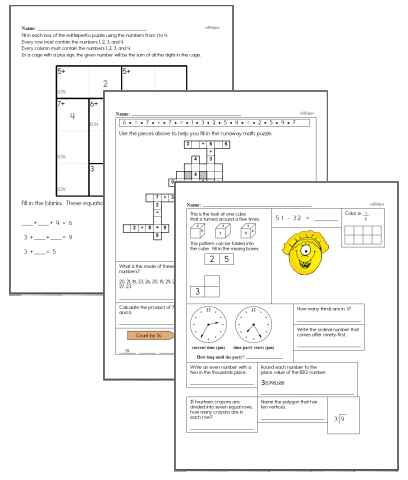Monthly Math Challenge Workbooks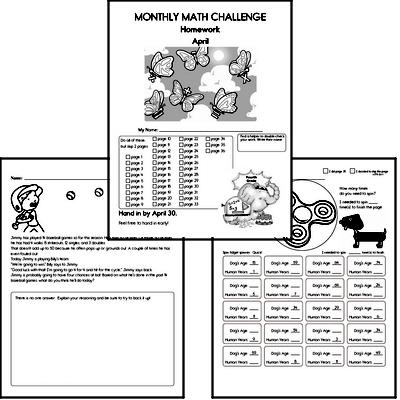4th Grade Math Challenge Book

Mental Math Workbooks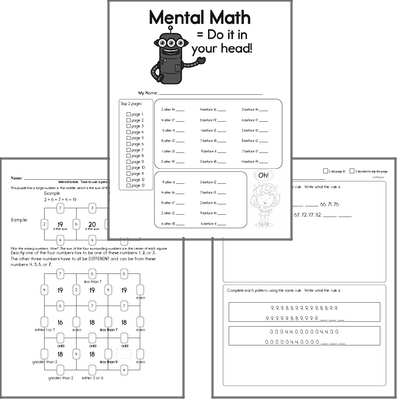Mental Math Workbooks

Fourth Grade Math Worksheets Activity Workbook - No Prep and Not Boring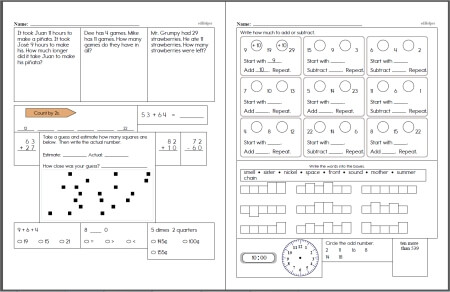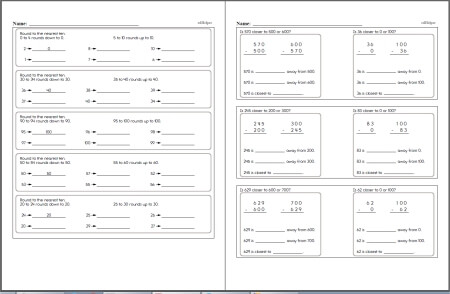Fourth Grade Math Book New Fourth Grade Math Workbook Created Each book contains word problems, mixed math, and other fourth grade review materials. Use it each week for daily practice in your classroom.

Daily Fourth Grade Math and Critical Thinking Practice

Fourth Grade Daily Homework and Practice Pages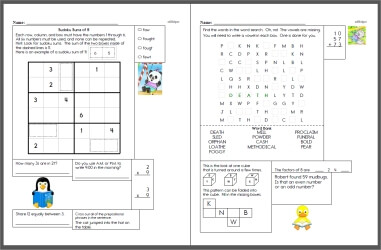Fourth Grade Daily Homework

Fourth Grade Mixed Review
Level 1: Easiest (First 2 months of school)
Level 2: Next 3 months of school
Level 3: Next 3 months of school
Level 4: Entire Grade (Final months of school)

Weekly Word Problems
Last week's fourth grade math word problems
This week's fourth grade math word problems
Next week's fourth grade math word problems
Daily Review: Customize math review skills with word problems, language arts, and science

edHelperKu Puzzles

Runaway Math Puzzles
 Fourth grade runaway math puzzles Runaway Math PuzzlesEquation Unknowns Puzzle

Build a Mixed Review Worksheet!
For edHelper.com subscribers. - Sign up now by clicking here!

Select the skills to include
Whole Numbers
 Place Value: Numbers to 999,999 Rounding Whole Numbers

Money
 Money

Addition, Subtraction, and Multiplication

Probability
 Probability

Number Theory
 Number Theory

Division
 Division Facts Through 10 Division by One-Digit Numbers Division by Two-Digit Numbers

Graph and Analyze Data
 Graph and Analyze Data Mean, Median, and Mode

Algebraic Reasoning
 Algebraic Reasoning

Geometry
 Plane Figures Area and Perimeter

Fractions
 Understanding Fractions Add and Subtract Like Fractions Add and Subtract Fractions

Decimals
Understanding Decimals
Add and Subtract Decimals

Number of Pages (for each key)
 Select the number of pages: 1 page 2 pages 3 pages 4 pages 5 pages 6 pages

Number of Keys
 Select the number of different printables: 1 key 2 keys 3 keys

Answer key also includes questions

Build a Custom Word Story Worksheet!
For edHelper.com subscribers. - Sign up now by clicking here!
Select the skills to include:
 Addition Subtraction Multiplication Division Time Decimals Fractions Percents and Ratios

 Select the number of word problems: 2 Word Problems 4 Word Problems 6 Word Problems

Number of Keys
 Select the number of different printables: 1 key 2 keys 3 keys

If more than 1 key:
Mix up the questions on each key

(optional)  Enter a class name or header:

Basic Operations
Subtraction
Multiplication
Division

Math Facts

Quick Math Facts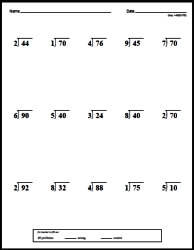Make Quick Math Facts Printable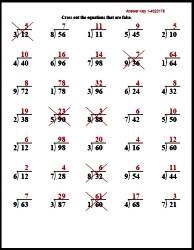Super Silly Squares

Subtraction Squares

Place Value

Rounding Whole Numbers
Round to the nearest ten
Round to the nearest hundred
Round to the nearest thousand
Write the 2 tens between each number
Write the 2 hundreds between each number
Write the 2 thousands between each number
Round to the place of the underlined digit (tens, hundreds, and thousands)
Fill in the missing digit (ones, tens, or hundreds digits blank)
Fill in the missing digit (tens, hundreds, or thousands digits blank)
Fill in the missing digit (hundreds, thousands, or ten-thousands digits blank)
Round to the nearest ten-thousand
Round to the nearest hundred-thousand
Round to the nearest million
Round to the place of the underlined digit (ten-thousands, hundred-thousands, and millions)
Round to the place of the underlined digit (ones through millions)
Rounding Whole Numbers Mixed Review

Money
Write in decimal form (money graphics)
Write in decimal form (sixty-five dollars and one cent)
Write in decimal form (4 one-dollar bills, 3 quarters, 1 dime)
Compare (money graphics)
Compare
Show amount using fewest number of coins (20 to 99 cents)
Show amount using fewest number of coins (20 to 99 cents; fill-ins have mixed order)
Show amount using fewest number of coins (\$0.20 to \$4.99)
Show amount using fewest number of coins (\$0.20 to \$19.99)
Make change using the given number of coins (20 to 99 cents)
Make change using the given number of coins (\$0.20 to \$4.99)
Make change using the given number of coins (\$0.20 to \$19.99)
Compare (\$1.59 ____ \$1.95)
Round to the nearest dollar
Round to the nearest ten dollars
Round to the place of the underlined digit
Problem solving
Money Mixed Review

2-3 digits column addition
2-4 digits column addition
4-7 digits column addition
2 digits across addition
2-4 digits across addition
4-5 digits across addition
2 digits with 3 numbers column addition
2-3 digits with 3 numbers column addition
2-5 digits with 3 numbers column addition
Column addition: start with 2 digits and increase by 1 digit in each row
Column addition: start with 2-3 digits and increase by 1 digits every 2 rows
Column addition: start with 2-3 digits and increase by 2 digits every 2 rows
Across addition with 3-5 numbers
Fill in the missing numbers: 2 digits
Fill in the missing numbers: 2-3 digits
Fill in the missing numbers: 4-5 digits
Fill in the missing numbers: 2-6 digits
Math word puzzles
Math addition boxes: 3 x 3
Math addition boxes: 4 x 4
Addition Word Problems (Easier)

Number Bonds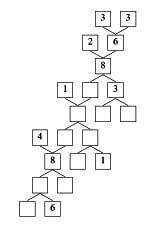Number Bonds

Subtraction
2 digits column subtraction
3 digits column subtraction
2-3 digits column subtraction
4 digits column subtraction
5-6 digits column subtraction
3-5 digits column subtraction
2 digits across subtraction
2-3 digits across subtraction
2-4 digits across subtraction
3-4 digits across subtraction
5-6 digits across subtraction
Column subtraction: start with 2 digits and increase by 1 digit in each row
Column subtraction: start with 2-3 digits and increase by 1 digits every 2 rows
Column subtraction: start with 2-3 digits and increase by 2 digits every 2 rows
Across subtraction with 3-5 numbers
Fill in the missing numbers: 2 digits
Fill in the missing numbers: 2-3 digits
Fill in the missing numbers: 4-5 digits
Fill in the missing numbers: 2-6 digits
Math word puzzles
Math addition boxes: 3 x 3
Math addition boxes: 4 x 4
Subtraction word problems (Easier)
Subtraction word problems
Subtraction Mixed Review

Multiplication
Column Multiplication with Extra Space To Solve Problems
1-2 digits by 1 digit column multiplication
2 digits (and increasing 1 digit every 2 rows) by 1 digit column multiplication
2 digits (and increasing 1 digit every row) by 1 digit column multiplication
Multiples of 10 multiplication (2-5 digits by 1 digit)
2 digits by 2 digits column multiplication
2-3 digits by 2 digits column multiplication
4-5 digits by 2 digits column multiplication
2 digits (and increasing 1 digit every 2 rows) by 2 digit column multiplication
2 digits (and increasing 1 digit every row) by 2 digit column multiplication

Column Multiplication (more problems with less space)
1-2 digits by 1 digit column multiplication
2 digits (and increasing 1 digit every 2 rows) by 1 digit column multiplication
2 digits (and increasing 1 digit every row) by 1 digit column multiplication
Multiples of 10 multiplication (2-5 digits by 1 digit)
2 digits by 2 digits column multiplication
2-3 digits by 2 digits column multiplication
4-5 digits by 2 digits column multiplication
2 digits (and increasing 1 digit every 2 rows) by 2 digit column multiplication
2 digits (and increasing 1 digit every row) by 2 digit column multiplication

Part 2
1-2 digits by 1 digit across multiplication
3-4 digits by 1 digit across multiplication
1-2 digits by 2 digits across multiplication
Across multiplication with 3-5 numbers
Fill in the missing numbers: 1-2 digits
Fill in the missing numbers: 2 digits
Fill in the missing numbers: 2-3 digits
Math multiplication boxes: 3 x 3 (numbers 1-9)
Math multiplication boxes: 4 x 4 (numbers 1-9)
Math multiplication boxes: 3 x 3 (numbers 4-19)
Multiplication word problems (basic)
Multiplication word problems
Multiplication word problems
Multiplication Mixed Review

Division Facts Through 10
Multiplication and Division: Fill in the missing numbers
Multiplication and division with 2
Multiplication and division with 3
Multiplication and division with 4
Multiplication and division with 5
Multiplication and division with 6
Multiplication and division with 7
Multiplication and division with 8
Multiplication and division with 9
Multiplication and division with 10
Multiplication and division (mix)
Division Facts
Divide by 2
Divide by 3
Divide by 4
Divide by 5
Divide by 6
Divide by 7
Divide by 8
Divide by 9
Divide by 10
Division (mix)
Division Facts
Division by 2 to 10
Division by 2 to 10
Fill in the missing number
Division Problem Solving
Division problem solving
Division Mixed Review
Division Facts Mixed Review

Division by One-Digit Numbers
Column Division with Extra Space To Solve Problems - Division by One-Digit Numbers
2 digits by 1 digit column division (no remainders)
2-3 digits by 1 digit column division (no remainders)
4-5 digits by 1 digit column division (no remainders)
5-7 digits by 1 digit column division (no remainders)
2 digits by 1 digit column division
2-3 digits by 1 digit column division
4-5 digits by 1 digit column division
5-7 digits by 1 digit column division
2 digits (and increasing 1 digit every 2 rows) by 1 digit column division
2 digits (and increasing 1 digit every row) by 1 digit column division

Column Division (more problems with less space) - Division by One-Digit Numbers
2 digits by 1 digit column division (no remainders)
2-3 digits by 1 digit column division (no remainders)
4-5 digits by 1 digit column division (no remainders)
5-7 digits by 1 digit column division (no remainders)
2 digits by 1 digit column division
2-3 digits by 1 digit column division
4-5 digits by 1 digit column division
5-7 digits by 1 digit column division
2 digits (and increasing 1 digit every 2 rows) by 1 digit column division
2 digits (and increasing 1 digit every row) by 1 digit column division

Part 2 - Division by One-Digit Numbers
Divisibility: complete the table
2 digits by 1 digit across division
2-3 digits by 1 digit across division
4-5 digits by 1 digit across division
2 digits (and increase by 1 digit every row) by 1 digit across division
Fill in the missing numbers: 2 digits by 1 digit
Fill in the missing numbers: 3 digits by 1 digit
Fill in the missing numbers: 4 digits by 1 digit
Division Word Problems
Solve equations: 2 digits by 1 digit
Order of operations: 2-3 digits by 1 digit (no parenthesis)
Order of operations: 2-3 digits by 1 digit
Order of operations: 2-4 digits by 1 digit
One-Digit Numbers Division Mixed Review

Division by Two-Digit Numbers
Column Division with Extra Space To Solve Problems - Division by Two-Digit Numbers
Division by multiples of 10: 3-4 digits by 2 digits (no remainders)
Division by multiples of 10: 5-7 digits by 2 digits (no remainders)
Division by multiples of 10: 3-4 digits by 2 digits
3-4 digits by 2 digits column division (no remainders)
4-5 digits by 2 digits column division (no remainders)
5-6 digits by 2 digits column division (no remainders)
6-7 digits by 2 digits column division (no remainders)
3-4 digits by 2 digits column division
4-5 digits by 2 digits column division
5-6 digits by 2 digits column division
6-7 digits by 2 digits column division
3 digits (and increasing 1 digit every 2 rows) by 2 digits column division
3 digits (and increasing 1 digit every row) by 2 digits column division

Column Division (more problems with less space) - Division by Two-Digit Numbers
Division by multiples of 10: 3-4 digits by 2 digits (no remainders)
Division by multiples of 10: 5-7 digits by 2 digits (no remainders)
Division by multiples of 10: 3-4 digits by 2 digits
3-4 digits by 2 digits column division (no remainders)
4-5 digits by 2 digits column division (no remainders)
5-6 digits by 2 digits column division (no remainders)
6-7 digits by 2 digits column division (no remainders)
3-4 digits by 2 digits column division
4-5 digits by 2 digits column division
5-6 digits by 2 digits column division
6-7 digits by 2 digits column division
3 digits (and increasing 1 digit every 2 rows) by 2 digits column division
3 digits (and increasing 1 digit every row) by 2 digits column division

Part 2 - Division by Two-Digit Numbers
Divisibility: complete the table
3-4 digits by 2 digits across division
4-5 digits by 2 digits across division
5-6 digits by 2 digits across division
3 digits (and increase by 1 digit every row) by 2 digits across division
Fill in the missing numbers: 3 digits by 2 digits
Fill in the missing numbers: 4 digits by 2 digits
Fill in the missing numbers: 5 digits by 2 digits
Division Word Problems
Order of operations: 2-3 digits by 1 digit
Order of operations: 3 digits by 2 digits
Two-Digit Numbers Division Mixed Review

Ordered Pairs and the Coordinate Plane

Graph and Analyze Data
Tally table
Frequency table
Line plots
Stem-and-leaf plots
Vertical bar graphs
Vertical bar graphs (draw graph from table)
Horizontal bar graphs
Horizontal bar graphs (draw graph from table)
Ordered pairs: Write the ordered pair for each point
Ordered pairs: Find each ordered pair. Write the letter for the point named by the ordered pair.
Ordered pairs: Draw the ordered points on the grid. Label each point.
Line graphs
Line graphs (draw graph from table)
Graph and Analyze Data Mixed Review
Stem-and-leaf plots (more difficult)
Vertical bar graphs (more difficult)
Horizontal bar graphs (more difficult)
Graph and Analyze Data Mixed Review

Mean, Median, and Mode

Line Plots
Calculating range from a line plot
Calculating mode from a line plot
Calculating median from a line plot
Calculating mean from a line plot
Mixed

Stem-and-Leaf Plots
Calculating range from a stem-and-leaf plots
Calculating mode from a stem-and-leaf plots
Calculating median from a stem-and-leaf plots
Calculating mean from a stem-and-leaf plots
Mixed

5-7 Numbers
Find the range
Find the mean
Find the median
Find the mode
Find the mean, median, and mode
Find the value
Fine the missing number

8-13 Numbers
Find the range
Find the mean
Find the median
Find the mode
Find the mean, median, and mode
Find the value
Fine the missing number

Review
Mean, Median, and Mode Mixed Review

Algebraic Reasoning
Order of Operations (3-4 numbers, no parenthesis)
Order of operations: simplify
Order of operations: fill in the missing number (1 missing)
Order of operations: fill in the missing operation (1 missing)
Order of operations: fill in all of the numbers
Order of operations: fill in all of the operations
Order of Operations (3-5 digits)
Order of operations: simplify
Order of operations: fill in the missing number (1 missing)
Order of operations: fill in the missing operation (1 missing)
Order of operations: fill in all of the numbers
Order of operations: fill in all of the operations
Algebraic Expressions
Evaluate expressions
More evaluate expressions
Writing algebraic expressions
Solving Equations
Solving subtraction equations
Solving multiplication and division equations
Solving addition, subtraction, multiplication, and division equations
Write an equation for each problem. Then solve the equation.
Functions
Complete the function table (rule and easier inputs given)
Complete the function table (rule and inputs given)
Complete the function table (missing some inputs and some outputs)
Write the rule for the function (all the inputs and outputs given)
Write the rule and fill in missing inputs or outputs
Algebraic Expressions Mixed Review
Algebraic Expressions Mixed Review

Plane Figures
Name each figure using letters and symbols
Draw and label an example of each
Classify each pair of lines as parallel, intersecting, or perpendicular
Classify each angle as acute, obtuse, straight, or right
Classify polygons
Classify triangles
Classify triangles according to lengths
Circles
Plane Figures Mixed Review

Slides, Turns, and Flips

Perimeter

Area and Perimeter
Find the perimeter of each figure
Find the perimeter of each figure using a ruler
Perimeter: Find the value of n
Find the area of each figure
Find the value of n
Find the area and perimeter of each rectangle (no graphics)
Find the perimeter of a complex polygon (composed of 2 rectangles)
Find the perimeter of a complex polygon (composed of 3 rectangles)
Find the perimeter of a complex polygon (composed of 4 rectangles)
Find the area and perimeter of a complex polygon (composed of 2 rectangles)
Find the area and perimeter of a complex polygon (composed of 3 rectangles)
Find the area and perimeter of a complex polygon (composed of 4 rectangles)
Area and Perimeter Mixed Review

Number Theory
Divisibility: circle all of the numbers that are divisible by the number
Divisibility: complete the table
Prime or composite
Use the clue to fill in the missing digit
Circle all of the numbers that are multiples of a number
List 3 multiples of each number
Fill in the missing multiple
List all of the factors
Prime factorization
Greatest common factor
Least common multiple
Number theory review printable

Understand Fractions
Circle the equivalent fraction
Write two equivalent fractions
Is each fraction in simplest form?
Write each fraction in simplest form
Equivalent fractions: fill in the missing number (easier)
Equivalent fractions: fill in the missing number
Comparing fractions
Ordering fractions (from least to greatest)
Ordering fractions (from greatest to least)
Part of a number
Problem solving
Circle the equivalent improper fraction
Circle the equivalent mixed number
Write each improper fraction as a mixed number
Write each mixed number as an improper fraction
Understand Fractions Mixed Review

Add and Subtract Fractions
Add Fractions with Like Denominators
Add fractions with like denominators (horizontal)
Add fractions with like denominators (vertical)
Add fractions with like denominators: find the value of y
Subtract Fractions with Like Denominators
Subtract fractions with like denominators (horizontal)
Subtract fractions with like denominators (vertical)
Subtract fractions with like denominators: find the value of y
Add and Subtract Fractions with Like Denominators
Add and subtract fractions with like denominators (horizontal)
Add and subtract fractions with like denominators (vertical)
Add and subtract fractions with like denominators: find the value of y
Add and Subtract Fractions with Like Denominators (Mixed Numbers)
Add and subtract fractions with like denominators and mixed numbers (horizontal)
Add and subtract fractions with like denominators and mixed numbers (vertical)
Add and subtract fractions with like denominators and mixed numbers: find the value of y
Add Fractions with Unlike Denominators
Add fractions with unlike denominators (horizontal)
Add fractions with unlike denominators (vertical)
Add fractions with unlike denominators: find the value of y
Subtract Fractions with Unlike Denominators
Subtract fractions with unlike denominators (horizontal)
Subtract fractions with unlike denominators (vertical)
Subtract fractions with unlike denominators: find the value of y
Add and Subtract Fractions with Unlike Denominators
Add and subtract fractions with unlike denominators (horizontal)
Add and subtract fractions with unlike denominators (vertical)
Add and subtract fractions with unlike denominators: find the value of y
Add and Subtract Fractions with Unlike Denominators (Mixed Numbers)
Add and subtract fractions with unlike denominators and mixed numbers (horizontal)
Add and subtract fractions with unlike denominators and mixed numbers (vertical)
Add and subtract fractions with unlike denominators and mixed numbers: find the value of y
Problem Solving
Find parts of a number
Addition and subtraction of fractions
Addition and subtraction of fractions (mixed numbers)
Addition and subtraction of fractions (multi-step)
Addition and subtraction of fractions (more problems)
Review
Add and Subtract Fractions Mixed Review

Understanding Decimals
Tenths
Write each as a decimal (given: graphics, fractions, and word form)
Given graphics, write the decimal (decimals greater than 1)
Write each as a decimal (decimals greater than 1)
Write each as a fraction

Tenths and Hundredths
Hundredths: Write each as a fraction and decimal
Hundredths: Write each as a decimal
Hundredths: Write each as a fraction
Tenths and hundredths: Write each as a decimal
Tenths and hundredths: Write each as a fraction
Hundredths: Write each as a decimal (graphics)
Tenths and hundredths greater than 1: Write each as a decimal
Tenths and hundredths greater than 1: Write each as a fraction
Tenths and hundredths: Write each as a decimal (mix: less than and greater than 1 decimals)
Tenths and hundredths: Write each as a fraction (mix: less than and greater than 1 decimals)
Tenths and hundredths: Compare decimals
Tenths and hundredths: Order decimals
Order decimals (from 6 to 8 numbers each)
Compare decimals and fractions
Order decimals and fractions
Order decimals and fractions (from 7 to 11 numbers each)
Compare decimals and fractions (with numbers greater than 1)
Order decimals and fractions (with numbers greater than 1)
Tenths and Hundredths Mixed Review

Thousandths
Thousandths: Write as a decimal
Thousandths: Write as a fraction
Thousandths: Write as a decimal (decimals greater than 1)
Thousandths: Write as a fraction (decimals greater than 1)

Tenths, Hundredths, and Thousandths
Write as a decimal
Write as a fraction
Compare decimals
Ordering decimals
Compare decimals (including fractions)
Ordering decimals (including fractions)

Rounding Decimals
Rounding: Round to the nearest tenth
Rounding: Round to the nearest hundredth
Rounding: Round to the place of the underlined digit

Review
Mixed Review

Add and Subtract Decimals
Column addition of 2 numbers (up to tenths)
Column addition of 2 numbers (up to hundredths)
Column addition of 2 numbers (up to thousandths)
Money column addition (up to \$99.99)
Money column addition (up to \$99,999.99)
Across addition of 2 numbers (up to hundredths)
Across addition of 2 numbers (up to thousandths)

Subtraction
Column subtraction of 2 numbers (up to tenths)
Column subtraction of 2 numbers (up to hundredths)
Column subtraction of 2 numbers (up to thousandths)
Money column subtraction (up to \$99.99)
Money column subtraction (up to \$99,999.99)
Across subtraction of 2 numbers (up to hundredths)
Across subtraction of 2 numbers (up to thousandths)

Mix of Addition and Subtraction
Column addition and subtraction of 2 numbers (up to tenths)
Column addition and subtraction of 2 numbers (up to hundredths)
Column addition and subtraction of 2 numbers (up to thousandths)
Money column addition and subtraction (up to \$99.99)
Money column addition and subtraction (up to \$99,999.99)
Across addition and subtraction of 2 numbers (up to hundredths)
Across addition and subtraction of 2 numbers (up to thousandths)

Part 2
Column addition of 3 numbers
Order of Operations (no parenthesis)
Order of Operations
Order of Operations (one missing number)
Order of Operations (one missing operation)
Order of Operations (fill in all numbers)
Order of Operations (fill in all operations)
Fill in the missing numbers (up to hundredths)
Fill in the missing numbers (up to thousandths)
Math box
Decimal addition and subtraction word problems
Addition and subtraction of decimals: Mixed Review

Test Prep - Concepts
from the test prep section, requires registration
Numeration
Number Concepts
Properties
Fractions and Decimals
Review of Number Concepts

Test Prep - Computation
from the test prep section, requires registration
Subtraction
Multiplication
Division
Review of Computation

Test Prep - Applications
from the test prep section, requires registration
Geometry
Measurement
Problem Solving - Word Problems!
Problem Solving Applications (Charts, etc)

Have a suggestion or would like to leave feedback?